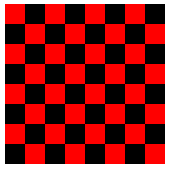On-line GuidesAll GuideseBook StoreiOS / AndroidLinux for BeginnersOffice ProductivityLinux InstallationLinux SecurityLinux UtilitiesLinux VirtualizationLinux KernelSystem/Network AdminProgrammingScripting LanguagesDevelopment ToolsWeb DevelopmentGUI Toolkits/DesktopDatabasesMail SystemsopenSolarisEclipse DocumentationTechotopia.comVirtuatopia.comAnswertopia.comHow To GuidesVirtualizationGeneral System AdminLinux SecurityLinux FilesystemsWeb ServersGraphics & DesktopPC HardwareWindowsProblem SolutionsPrivacy Policy### Solution for Programming Exercise 3.5

THIS PAGE DISCUSSES ONE POSSIBLE SOLUTION to the following exercise from this on-line Java textbook.

Exercise 3.5: Write an applet that draws a checkerboard. Assume that the size of the applet is 160 by 160 pixels. Each square in the checkerboard is 20 by 20 pixels. The checkerboard contains 8 rows of squares and 8 columns. The squares are red and black. Here is a tricky way to determine whether a given square is red or black: If the row number and the column number are either both even or both odd, then the square is red. Otherwise, it is black. Note that a square is just a rectangle in which the height is equal to the width, so you can use the subroutine g.fillRect() to draw the squares. Here is an image of the checkerboard:(To run an applet, you need a Web page to display it. A very simple page will do. Assume that your applet class is called Checkerboard, so that when you compile it you get a class file named Checkerboard.class Make a file that contains only the lines:

```           <applet code="Checkerboard.class" width=160 height=160>
</applet>
```

Call this file Checkerboard.html. This is the source code for a simple Web page that shows nothing but your applet. You can open the file in a Web browser or with Sun's appletviewer program. The compiled class file, Checkerboard.class, must be in the same directory with the Web-page file, Checkerboard.html.)

Discussion

The basic algorithm is obvious:

```            for each row on the checkerboard:
Draw all the squares in that row
```

Since any given row contains eight squares, one in each column of the checkerboard, we can expand the body of the for loop into another for loop:

```            for each row on the checkerboard:
for each of the eight columns:
Draw the square in that row and column
```

Each square is a rectangle with height 20 and width 20, so it can be drawn with the command g.fillRect(x,y,20,20), where x and y are the coordinates of the top-left corner of the square. Before drawing the square, we have to determine whether it should be red or black, and we have to set the correct color with g.setColor. So, the algorithm becomes

```            for each row on the checkerboard:
for each of the eight columns:
Compute x,y for the top-left corner of the square
if its a red square:
g.setColor(Color.red)
else
g.setColor(Color.black)
g.fillRect(x,y,20,20)
```

The top of the first row of squares is at y=0. Since each square is 20 pixels high, the top of the second row is at y=20, followed by 40 for the third row, then 60, 80, 100, 120, and 140. If we assume that the rows are numbered 0, 1, 2, ..., 7, then the tops are given by y = row*20, where row is the row number. (If you number the rows 1, 2, ..., 8, the formula would be (row-1)*20. The simpler formula in this and in many similar cases is one reason why computer scientists like to start counting with 0 instead of 1.) Similarly, the left edge of the squares in column col is given by x = col*20, where again the columns are numbered 0, 1, 2, ..., 7. I'll use "for (row=0; row<8; row++)" to count off the rows, rather than the equivalent "for (row=0; row<=7; row++)". The 8 reminds me that I am counting off the eight numbers 0, 1, 2, ..., 7. Again, this is typical computer science style.

The only problem remaining is how to determine whether the square is red. As noted in the exercise, a square is red if row and col are either both even or both odd. Since an integer N is even if N%2 is 0, the test could be expressed as "if ((row%2 == 0 && col%2 == 0) || (row%2 == 1 && col%2 == 1))". However, note that this is the same as asking whether row%2 and col%2 have the same value. So the test can be written more simply as "if (row%2 == col%2)". Putting this all together into syntactically correct Java code, the algorithm becomes

```            for ( row = 0;  row < 8;  row++ ) {
for ( col = 0;  col < 8;  col++ ) {
x = 20*col;
y = 20*row;
if ( (row % 2) == (col % 2) )
g.setColor(Color.red);
else
g.setColor(Color.black);
g.fillRect(x,y,20,20);
}
}
```

Of course, the variables row, col, x, and y have to be declared to be of type int. Then, the code goes into the body of the paint() method of an applet. The syntax of the applet definition must follow the format given in Section 3.7.

The Solution

```    import java.awt.*;
import java.applet.*;
public class Checkerboard extends Applet {
/*  This applet draws a red-and-black checkerboard.
It is assumed that the size of the applet is 160
by 160 pixels.
*/
public void paint(Graphics g) {
int row;   // Row number, from 0 to 7
int col;   // Column number, from 0 to 7
int x,y;   // Top-left corner of square
for ( row = 0;  row < 8;  row++ ) {
for ( col = 0;  col < 8;  col++) {
x = col * 20;
y = row * 20;
if ( (row % 2) == (col % 2) )
g.setColor(Color.red);
else
g.setColor(Color.black);
g.fillRect(x, y, 20, 20);
}
} // end for row
}  // end paint()
}  // end class
```

[ Exercises | Chapter Index | Main Index ]Published under free license. Design by Interspire